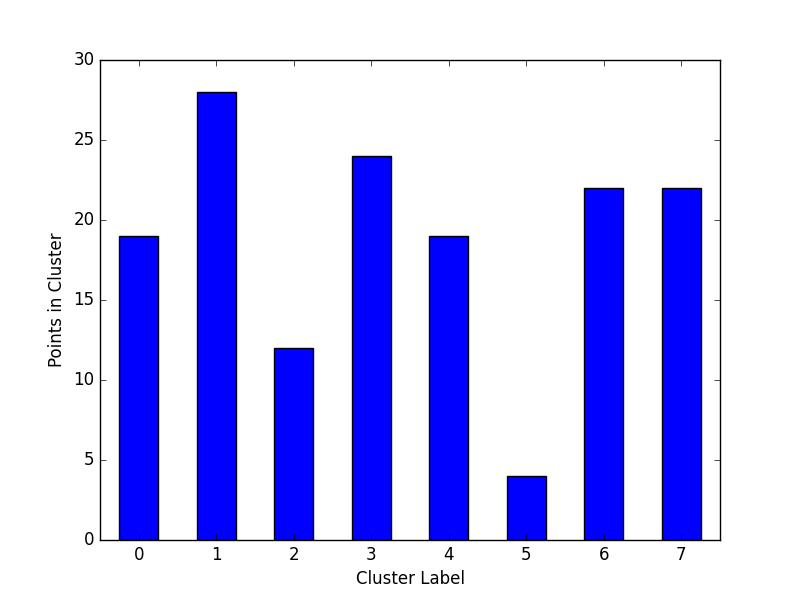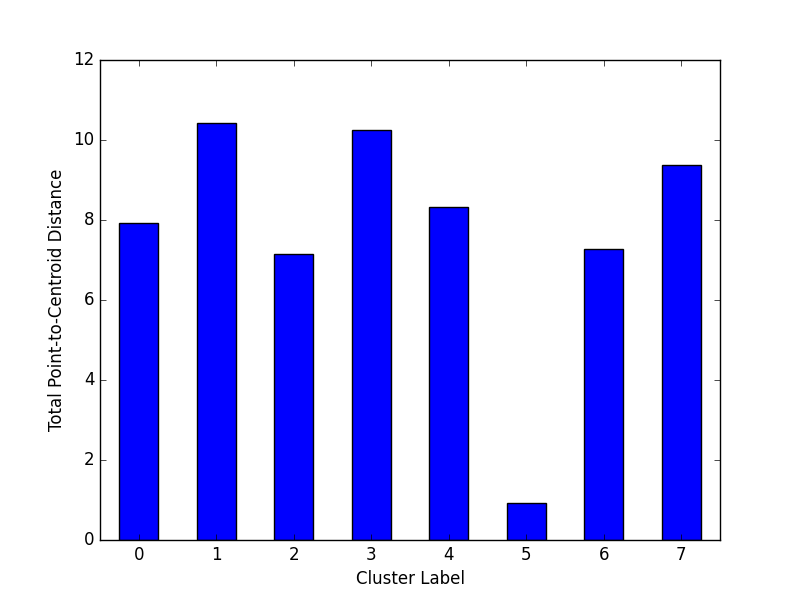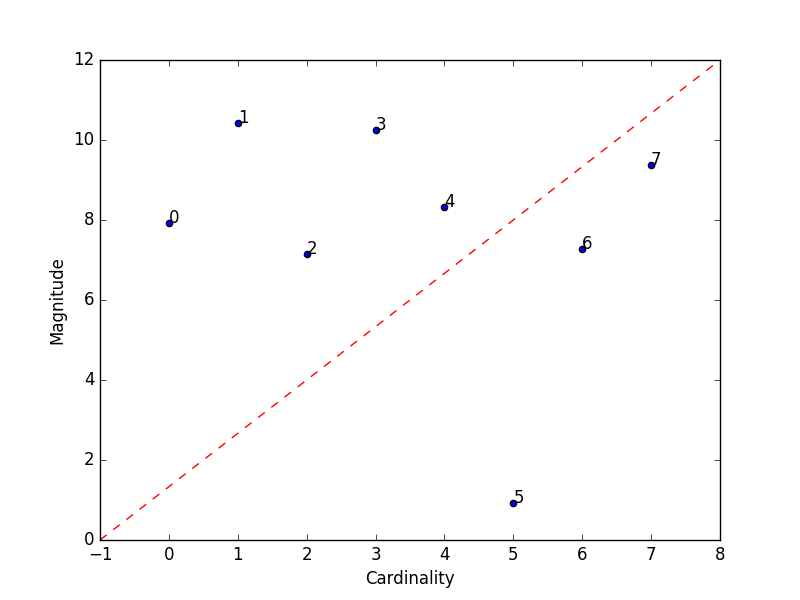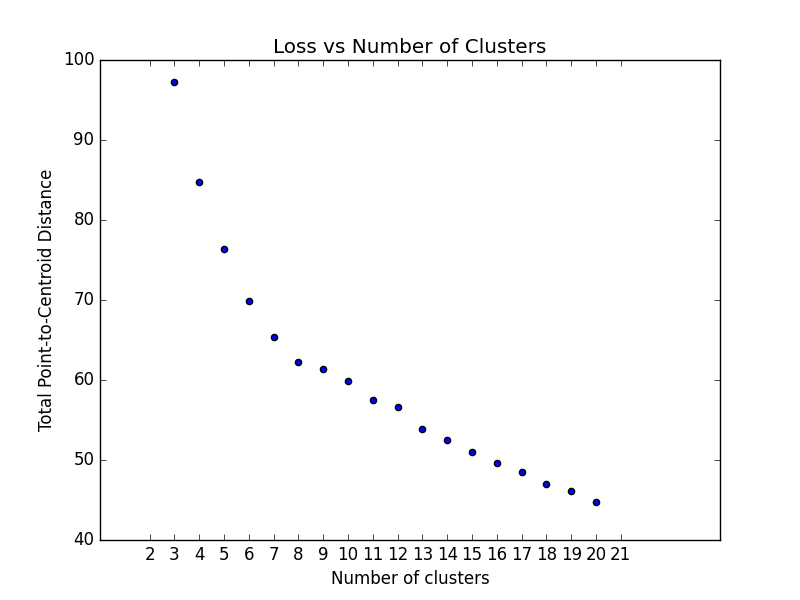This project is an ensemble of methods which are frequently used in python Data Science projects.

# Data Science Utils: Frequently Used Methods for Data ScienceData Science Utils extends the Scikit-Learn API and Matplotlib API to provide simple methods that simplify task and visualization over data.

# Code Examples and Documentation

Let's see some code examples and outputs.

You can read the full documentation with all the code examples from: https://datascienceutils.readthedocs.io/en/latest/

In the documentation you can find more methods and more examples.

The API of the package is build to work with Scikit-Learn API and Matplotlib API. Here are some of capabilities of this package:

## Metrics

### Plot Confusion Matrix

Computes and plot confusion matrix, False Positive Rate, False Negative Rate, Accuracy and F1 score of a classification.

from ds_utils.metrics import plot_confusion_matrix

plot_confusion_matrix(y_test, y_pred, [0, 1, 2])### Plot Metric Growth per Labeled Instances

Receives a train and test sets, and plots given metric change in increasing amount of trained instances.

from ds_utils.metrics import plot_metric_growth_per_labeled_instances

plot_metric_growth_per_labeled_instances(x_train, y_train, x_test, y_test,
{"DecisionTreeClassifier":
DecisionTreeClassifier(random_state=0),
"RandomForestClassifier":
RandomForestClassifier(random_state=0, n_estimators=5)})### Visualize Accuracy Grouped by Probability

Receives test true labels and classifier probabilities predictions, divide and classify the results and finally plots a stacked bar chart with the results. Original code

from ds_utils.metrics import visualize_accuracy_grouped_by_probability

visualize_accuracy_grouped_by_probability(test["target"], 1,
classifier.predict_proba(test[selected_features]),
display_breakdown=False)


Without breakdown:With breakdown:## Preprocess

### Visualize Feature

Receives a feature and visualize its values on a graph:

• If the feature is float then the method plots the distribution plot.
• If the feature is datetime then the method plots a line plot of progression of amount thought time.
• If the feature is object, categorical, boolean or integer then the method plots count plot (histogram).
from ds_utils.preprocess import visualize_feature

visualize_feature(X_train["feature"])

Feature Type Plot
FloatIntegerDatetimeCategory / ObjectBoolean### Get Correlated Features

Calculate which features correlated above a threshold and extract a data frame with the correlations and correlation to the target feature.

from ds_utils.preprocess import get_correlated_features

correlations = get_correlated_features(train, features, target)

level_0 level_1 level_0_level_1_corr level_0_target_corr level_1_target_corr
income_category_Low income_category_Medium 1.0 0.1182165609358650 0.11821656093586504
term_ 36 months term_ 60 months 1.0 0.1182165609358650 0.11821656093586504
interest_payments_High interest_payments_Low 1.0 0.1182165609358650 0.11821656093586504

### Visualize Correlations

Compute pairwise correlation of columns, excluding NA/null values, and visualize it with heat map. Original code

from ds_utils.preprocess import visualize_correlations

visualize_correlations(data)### Plot Correlation Dendrogram

Plot dendrogram of a correlation matrix. This consists of a chart that that shows hierarchically the variables that are most correlated by the connecting trees. The closer to the right that the connection is, the more correlated the features are. Original code

from ds_utils.preprocess import plot_correlation_dendrogram

plot_correlation_dendrogram(data)### Plot Features' Interaction

Plots the joint distribution between two features:

• If both features are either categorical, boolean or object then the method plots the shared histogram.
• If one feature is either categorical, boolean or object and the other is numeric then the method plots a boxplot chart.
• If one feature is datetime and the other is numeric or datetime then the method plots a line plot graph.
• If one feature is datetime and the other is either categorical, boolean or object the method plots a violin plot (combination of boxplot and kernel density estimate).
• If both features are numeric then the method plots scatter graph.
from ds_utils.preprocess import plot_features_interaction

plot_features_interaction("feature_1", "feature_2", data)

Numeric Categorical Boolean Datetime
NumericCategoricalBooleanDatetime## Strings

### Append Tags to Frame

Extracts tags from a given field and append them as dataframe.

A dataset that looks like this:

x_train:

article_name article_tags
1 ds,ml,dl
2 ds,ml

x_test:

article_name article_tags
3 ds,ml,py

Using this code:

import pandas

from ds_utils.strings import append_tags_to_frame

x_train = pandas.DataFrame([{"article_name": "1", "article_tags": "ds,ml,dl"},
{"article_name": "2", "article_tags": "ds,ml"}])
x_test = pandas.DataFrame([{"article_name": "3", "article_tags": "ds,ml,py"}])

x_train_with_tags, x_test_with_tags = append_tags_to_frame(x_train, x_test, "article_tags", "tag_")


will be parsed into this:

x_train_with_tags:

article_name tag_ds tag_ml tag_dl
1 1 1 1
2 1 1 0

x_test_with_tags:

article_name tag_ds tag_ml tag_dl
3 1 1 0

### Extract Significant Terms from Subset

Returns interesting or unusual occurrences of terms in a subset. Based on the elasticsearch significant_text aggregation.

import pandas

from ds_utils.strings import extract_significant_terms_from_subset

corpus = ['This is the first document.', 'This document is the second document.',
'And this is the third one.', 'Is this the first document?']
data_frame = pandas.DataFrame(corpus, columns=["content"])
# Let's differentiate between the last two documents from the full corpus
subset_data_frame = data_frame[data_frame.index > 1]
terms = extract_significant_terms_from_subset(data_frame, subset_data_frame,
"content")


And the following table will be the output for terms:

third one and this the is first document second
1.0 1.0 1.0 0.67 0.67 0.67 0.5 0.25 0.0

## Unsupervised

### Cluster Cardinality

Cluster cardinality is the number of examples per cluster. This method plots the number of points per cluster as a bar chart.

import pandas
from matplotlib import pyplot
from sklearn.cluster import KMeans

from ds_utils.unsupervised import plot_cluster_cardinality

estimator = KMeans(n_clusters=8, random_state=42)
estimator.fit(data)

plot_cluster_cardinality(estimator.labels_)

pyplot.show()### Plot Cluster Magnitude

Cluster magnitude is the sum of distances from all examples to the centroid of the cluster. This method plots the Total Point-to-Centroid Distance per cluster as a bar chart.

import pandas
from matplotlib import pyplot
from sklearn.cluster import KMeans
from scipy.spatial.distance import euclidean

from ds_utils.unsupervised import plot_cluster_magnitude

estimator = KMeans(n_clusters=8, random_state=42)
estimator.fit(data)

plot_cluster_magnitude(data, estimator.labels_, estimator.cluster_centers_, euclidean)

pyplot.show()### Magnitude vs. Cardinality

Higher cluster cardinality tends to result in a higher cluster magnitude, which intuitively makes sense. Clusters are anomalous when cardinality doesn't correlate with magnitude relative to the other clusters. Find anomalous clusters by plotting magnitude against cardinality as a scatter plot.

import pandas
from matplotlib import pyplot
from sklearn.cluster import KMeans
from scipy.spatial.distance import euclidean

from ds_utils.unsupervised import plot_magnitude_vs_cardinality

estimator = KMeans(n_clusters=8, random_state=42)
estimator.fit(data)

plot_magnitude_vs_cardinality(data, estimator.labels_, estimator.cluster_centers_, euclidean)

pyplot.show()### Optimum Number of Clusters

k-means requires you to decide the number of clusters k beforehand. This method runs the KMean algorithm and increases the cluster number at each try. The Total magnitude or sum of distance is used as loss.

Right now the method only works with sklearn.cluster.KMeans.

import pandas

from matplotlib import pyplot
from scipy.spatial.distance import euclidean

from ds_utils.unsupervised import plot_loss_vs_cluster_number

plot_loss_vs_cluster_number(data, 3, 20, euclidean)

pyplot.show()## XAI

### Generate Decision Paths

Receives a decision tree and return the underlying decision-rules (or 'decision paths') as text (valid python syntax). Original code

from sklearn.tree import DecisionTreeClassifier

from ds_utils.xai import generate_decision_paths

# Create decision tree classifier object
clf = DecisionTreeClassifier(max_depth=3)

# Train model
clf.fit(x, y)
print(generate_decision_paths(clf, feature_names, target_names.tolist(),
"iris_tree"))


The following text will be printed:

def iris_tree(petal width (cm), petal length (cm)):
if petal width (cm) <= 0.8000:
# return class setosa with probability 0.9804
return ("setosa", 0.9804)
else:  # if petal width (cm) > 0.8000
if petal width (cm) <= 1.7500:
if petal length (cm) <= 4.9500:
# return class versicolor with probability 0.9792
return ("versicolor", 0.9792)
else:  # if petal length (cm) > 4.9500
# return class virginica with probability 0.6667
return ("virginica", 0.6667)
else:  # if petal width (cm) > 1.7500
if petal length (cm) <= 4.8500:
# return class virginica with probability 0.6667
return ("virginica", 0.6667)
else:  # if petal length (cm) > 4.8500
# return class virginica with probability 0.9773
return ("virginica", 0.9773)


## Plot Features Importance

plot feature importance as a bar chart.

import pandas

from matplotlib import pyplot
from sklearn.tree import DecisionTreeClassifier

from ds_utils.xai import plot_features_importance

target = data["target"]
features = data.columns.to_list()
features.remove("target")

clf = DecisionTreeClassifier(random_state=42)
clf.fit(data[features], target)
plot_features_importance(features, clf.feature_importances_)

pyplot.show()Excited?

• Metrics - The module of metrics contains methods that help to calculate and/or visualize evaluation performance of an algorithm.
• Preprocess - The module of preprocess contains methods that are processes that could be made to data before training.
• Strings - The module of strings contains methods that help manipulate and process strings in a dataframe.
• Unsupervised - The module od unsupervised contains methods that calculate and/or visualize evaluation performance of an unsupervised model.
• XAI - The module of xai contains methods that help explain a model decisions.

## Contributing

Interested in contributing to Data Science Utils? Great! You're welcome, and we would love to have you. We follow the Python Software Foundation Code of Conduct and Matplotlib Usage Guide.

No matter your level of technical skill, you can be helpful. We appreciate bug reports, user testing, feature requests, bug fixes, product enhancements, and documentation improvements.

## Find a Bug?

Check if there's already an open issue on the topic. If needed, file an issue.

## Installing Data Science Utils

Data Science Utils is compatible with Python 3.6 or later. The simplest way to install Data Science Utils and its dependencies is from PyPI with pip, Python's preferred package installer:

pip install data-science-utils


Note that this package is an active project and routinely publishes new releases with more methods. In order to upgrade Data Science Utils to the latest version, use pip as follows:

pip install -U data-science-utils


Alternatively you can install from source by cloning the repo and running:

git clone https://github.com/idanmoradarthas/DataScienceUtils.git
cd DataScienceUtils
python setup.py install


Or install using pip from source:

pip install git+https://github.com/idanmoradarthas/DataScienceUtils.git


If you're using Anaconda, you can install using conda:

conda install -c idanmorad data-science-utils


## Project details

This version1.7.11.71.6.31.6.21.6.11.61.51.4.11.4

Uploaded source
Uploaded py3`